11.  NEW THEORY OF LOW VOLTAGE ELECROLYSIS OF WATER

11.1. Contradictions  of the existing theory  of  water  electrolysis

American scientists Fleishmann and Pons have reported about obtaining of additional energy during water electrolysis in 1989 year and about the fact that cold nuclear fusion is the source of this energy . Hundreds of experiments were made in various countries in order to check this fact , , , , , , , , , . A part of the scientists confirmed it, and another part received a negative result , . The cause of such state remains unclear. That’s why let us analyse the water electrolysis process once again and try to make out with the essence of the processes, which take place .

The results of the given experiment put the main question: by which physical and chemical phenomenon is additional energy generated during usual water electrolysis? The first hypothesis: nuclear fusion at low temperature (cold nuclear fusion) is being checked from 1989 year, but still it has no definite confirmation , . The second hypothesis assumes that there is a certain particle called  “ersion”, which is responsible for the emergence of additional energy . But the main characteristics of this particles remain unknown, that’s why it is necessary to check one more hypothesis: additional energy is extracted from energies of chemical bonds of water molecules.

The main purpose of electrolysis of water is obtaining of  hydrogen, which is considered to be the most prospective energy carrier in the future , , , , . It has been discovered quite recently that in some cases access energy is released when this process takes place . If we want to find out its reason, we should analyses a classical method of hydrogen production by means of water electrolysis. It is described in reference books. Chemical reactions, which take place during this process, are used for the calculations of its parameters. They are considered to be clear and do not give rise to objections of both chemists  and physicists. Let us consider if it is actually clear.

Cathode and anode reactions are described in the manual in such a way . “The following reaction takes place at the cathode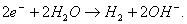(257)

Two electrons, which have arrived from the cathode, react with two molecules of water forming hydrogen molecule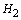and two ions of hydroxyl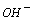. Molecular hydrogen forms the bubbles of gaseous hydrogen (when the solution near the cathode has been saturated with hydrogen), and the ions of hydroxyl remain in the solution.

The following reaction takes place at the anode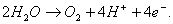(258)

Four electrons come to the anode from two molecules of water which is decomposed with the formation of an oxygen molecule and four ions of hydrogen.

Total reaction can be obtained if the equation (257) is multiplied by 2 and added together with the equation (258). It is put down in the following way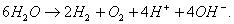(259)

… in acid solutions with a high concentration of hydrogen ions, the following reaction cam take place at the cathode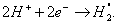(260)

Let us close quotation marks and put the following question: how should be the symbol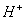interpreted in this description of the essence of electrolysis process? Naturally,means positive ion of hydrogen atom, i.e. proton. But the chemists have managed to designate a positively charged ion of hydroxonium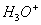with the same symbol. They have come to an agreement: to write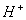instead of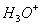for the purpose of simplification. We have nothing but guess: should we always understand a chemical symbolas a set of symbols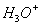or no? If so, how should we distinguish the cases “yes” and “no”? Symbolis used in many other chemical reactions. We should note that it is not the author of the above-mentioned manual who should be blamed, he has acted in the way as others act.

If we agree with the process of electrolysis described in the given manual, it means that water solution has protons, which are designated with the help of the symbolby the author. As they have a positive charge, they move to the cathode; having obtained electron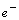from it, they form hydrogen atoms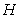, which, being connected, form the molecules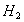. It is impossible to interpret the formula in another way (258) and (260).

Besides, it appears from the formula (258) that at the anode the fusion process of the oxygen molecules from its atoms takes place, which should be accompanied by release of energy of  495 kJ/mole . It should be taken into account during the analysis of energy balance of water electrolysis process, but in modern chemistry they are not accustomed to carry out such analysis, because it gives lots of contradictions with the experiment.

The first contradiction. Let us introduce symbolfor the designation of the proton only, as it should be. The hydrogen atom formation reaction is written in the following way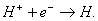(261)

The hydrogen molecule formation reaction is presented in the following way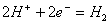.                                                                           (262)

The hypothesis concerning the presence of free protons in various chemical solutions is frequently used in chemistry. By the appearance of plasma electrolysis of water casts doubt on this hypothesis. The matter is that the proton is an active formation, which can exist only in the composition of various chemical compounds. If the conditions are created under which the proton is separated from the chemical compound, it lacks the existence phase in the free state almost completely. Having separated from one chemical element, it unites with other one at once.

Evidently there are such conditions when the proton of hydrogen atom having separated from water molecule is connected with the electron emitted by the cathode and forms hydrogen atom. It is known that hydrogen atoms exist at the temperature of 5000…10000°C . It means that plasma with such temperature should be formed at a certain density of hydrogen atoms in the unit of volume. As it is known, no atomic hydrogen plasma is formed during low-voltage electrolysis of water. It means that there is no atomic hydrogen fusion process in this case.

Hydrogen molecule fusion energy (436 kJ/mole) is known in modern chemistry, that’s why we can calculate approximate quantity of  energy, which should be released in electrolytic solution during production of one cubic meter of hydrogen , , .

One cubic meter of hydrogen contains 1000/22.4=44.64 mole of molecular hydrogen. Energy is released at its fusion.                                  (263)

Modern electrolyzers consume nearly 4 kWh of electric power or (3600 x 4)=14400 kJ for obtaining one cubic meter of hydrogen. Taking into consideration energy (19463.0) of fusion of one cubic meter of hydrogen and energy (14400) consumed by its production, we shall find water electrolysis process efficiency index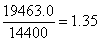(264)

Thus, a simple and rigorous calculation shows that the process of low voltage water electrolysis should be accompanied by a release of 35% of additional heat energy in the cathode area only.

Let us pay attention to the fact that this index takes into consideration only energy of the fusion of hydrogen molecules and does not take into account energy of the fusion of hydrogen atoms as well as energy content of produced hydrogen.

Efficiency index (264) confirms the possibility of obtaining of additional energy, but only under the condition that electrolysis of water is accompanied with fusion process of hydrogen molecules. Lack of additional energy in the operation of modern industrial electrolyzers makes us draw an absurd conclusion (on the face of it) concerning the lack of fusion process of hydrogen molecules from its atoms. Later on we’ll show that it is so indeed.

If we agree that there is a fusion process of the oxygen molecules, the following reaction should take place in the anode area: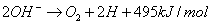,                                                        (265)

It means that the oxygen molecules and the hydrogen atoms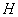should be formed. But, as it is known, only oxygen can be released in the anode area during low-voltage water electrolysis.

It is known that during low-voltage water electrolysis the process of formation of 1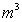(44.64 moles) of hydrogen is accompanied by a release of 22.32 moles of molecular oxygen. As a result, 495x22.32=11048.80 kJ of energy should be released. If we add this energy to hydrogen molecule fusion energy, we’ll get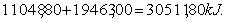(266)

Total index of energy efficacy of low-voltage process of water electrolysis should be as follows: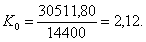(267)

It is known that heat energy index of modern low-voltage process of water electrolysis is less than unit. It means that there is no fusion of the molecules of hydrogen and oxygen in this process.

A question arises: how are the molecules of hydrogen and oxygen formed during low-voltage process of water electrolysis? Later on, we’ll show that the molecules of hydrogen and oxygen are released from the cluster chains in the formed conditions, i.e. without the bond process of separate atoms and molecules.

Thus, if chemical reactions (257) and (258) took place in modern electrolyser, they would have efficiency index more than one, ... as it follows from the ratio (264). The lack of additional energy during the low voltage process of water electrolysis is the result of formation of hydrogen molecules according to the diagram shown in Fig. 78.

The second contradiction. Now let us check the correspondence of the reality of binding energy of hydrogen atoms in water molecules accepted in modern chemistry. Modern chemistry theory gives values of binding energies of hydrogen atoms with oxygen atom in water molecule, but they differ from experimental values of these energies.

For example, in the book  in chemistry  the following value of binding energy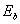of one hydrogen atom (let us call it the first one  -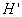) with oxygen atom (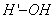) in water molecules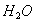=424 kJ per mole is given . Let us transform it into electron-volts calculating on one water molecule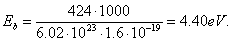(268)

Binding energy of the second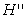hydrogen atom with oxygen atom (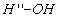) in water molecule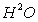is equal to=502 kJ per mole . In terms of electron-volts calculating on one bond we’ll have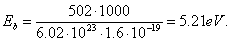(269)

If the hydrogen atoms in water molecule have binding energies corresponding to energies given in the formulas (268) and (269), cleavage of two bonds with energies of 4.40 eV should result in the formation of the hydrogen molecule; an oxygen molecule should be formed when two bonds with energies of 5.21 eV are broken. The energy expenses for the formation of one cubic meter of hydrogen and oxygen being obtained will be 424.00х44.64=18927.36 kJ and  502.00х 22.32= 11204.64  kJ, respectively. Total energy expenses for production of one cubic metre of hydrogen will be 18927.36+11204.64=30132.00 kJ or 30132.00/3600=8.37 kWh. It is twice as much of the experimental value. Consequently, the above-mentioned (268) and (269) binding energies of the hydrogen atoms with the oxygen atom in water molecule do not correspond to reality.

In which experiments are the above-mentioned binding energies registered? The experiments connected with water electrolysis and spectroscopy are the most congenial. Let us take minimal expenses of energy for hydrogen production established experimentally 3.8 kW×h per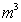. Let us represent this energy in terms of kJ. 3.8x3600=13680 kJ. Taking into account the fact that molar volume of all gases is similar and is equal to 22.4 L, we’ll find quantity of moles of molecular hydrogen in one cubic meter of hydrogen 1000/22.4=44.64 moles. Energy consumption per mole of hydrogen is 13680/44.64=306.45 kJ and per molecule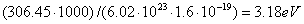.                                           (270)

In order to form the hydrogen molecule during water molecule destruction, at least two bonds of water molecules should be destroyed; it means that mean energy per bond is 3.18/2=1.59 eV. The value does not match the result given the formulas (266) and (267). If we take into account inevitable energy losses during electrolysis, we have every reason to believe that energy 1.59 eV is congenial to binding energies of the electron in hydrogen atom (Table 5) when it is on the third energy level (1.51 eV). It is congenital to binding energy of the eight electron in oxygen atom (1.53 eV) when it is on the similar energy level (Table 21). It results in almost equal probability of separation of both protons and hydrogen atoms from water molecules. The detailed analysis of this process will be given below.

Thus, the above-mentioned tables have no binding energies, which correspond to the existing calculations of chemists (266), (267); vice verse, these tables contain binding energies resulting from out calculation of the existing process of water electrolysis.

So, we have every reason to believe that the  electron in the first atom of hydrogen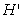in water molecule and the 1th electron in oxygen atom are on the third energy levels.

As it is clear, existing theoretical chemistry has serious contradictions with an experiment, but chemists avoid the search of the reason of these contradictions, they do not take into consideration the arising questions. The answers to them can be obtained from the analysis results of water molecule structure .

We’ll not find the answers to these questions within the framework of existing physical and chemical notions concerning the structure of water molecule and the process of its electrolysis, that’s why we have only one possibility: to pay attention to the own results of the investigations in this field , , , , , , , , , ,  and to take the water molecule structure, which originates from  the atomic nucleus structures, of hydrogen (Fig. 50, a) and oxygen (Fig. 61), as the basis.

The Foundations of Physchemistry of Microworld

Ó2003 Kanarev Ph. M.

Internet Version - http://book.physchemistry.innoplaza.net

<< Back to Physchemistry Book Index

 We’d like to remind that the value equal to the product of Avogadro number N=6.022×1023 and the electron charge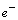=1.602×10-19  is Faraday constant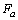. The value is measured in coulombs (C) per mole of the substance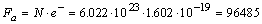C/mol.